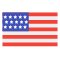enStyles
Categories
Trends
Old Interface
CollectionsView All
Drag icons
here

# Approximately equal Icons

Approximate
Collection
Approximate
Collection
Approximate
Collection
Approximately Not Equal
Collection
Approximately Not Equal
Collection
Approximately Not Equal
Collection
Approximately Not Equal
Collection
Approximately Not Equal
Collection
Approximately Not Equal
Collection
Approximately Not Equal
Collection
Approximately Not Equal
Collection
Approximately Not Equal
Collection
Approximately Not Equal
Collection
Approximately Not Equal
Collection
Approximately Not Equal
Collection
Approximately Not Equal
Collection
Approximately Not Equal
Collection
Approximately Not Equal
Collection
Approximately Not Equal
Collection
Approximately Not Equal
Collection
Approximately Not Equal
Collection
Approximately Not Equal
Collection
Approximately Not Equal
Collection
Approximately Not Equal
Collection
Approximately Not Equal
Collection
Approximately Not Equal
Collection
Approximately Not Equal
Collection
Approximately Not Equal
Collection
Approximately Not Equal
Collection
Approximately Not Equal
Collection
Approximately Not Equal
Collection
Approximately Not Equal
Collection
Approximately Not Equal
Collection
Approximately Not Equal
Collection
Approximately Not Equal
Collection
Approximately Not Equal
Collection
More or Equal
Collection
More or Equal
Collection
More or Equal
Collection
More or Equal
Collection
More or Equal
Collection
More or Equal
Collection
More or Equal
Collection
More or Equal
Collection
More or Equal
Collection
More or Equal
Collection
More or Equal
Collection
More or Equal
Collection
More or Equal
Collection
More or Equal
Collection
More or Equal
Collection
More or Equal
Collection
More or Equal
Collection
More or Equal
Collection
More or Equal
Collection
Less or Equal
Collection
Less or Equal
Collection
Less or Equal
Collection
Less or Equal
Collection
Less or Equal
Collection
Less or Equal
Collection
Less or Equal
Collection
Less or Equal
Collection
Less or Equal
Collection
Less or Equal
Collection
Less or Equal
Collection
Less or Equal
Collection
Less or Equal
Collection
Less or Equal
Collection
Less or Equal
Collection
Less or Equal
Collection
Less or Equal
Collection
Less or Equal
Collection
Less or Equal
Collection
Equal Sign
Collection
Equal Sign
Collection
Equal Sign
Collection
Equal Sign
Collection
Equal Sign
Collection
Equal Sign
Collection
Equal Sign
Collection
Equal Sign
Collection
Equal Sign
Collection
Equal Sign
Collection
Equal Sign
Collection
Equal Sign
Collection
Equal Sign
Collection
Equal Sign
Collection
Equal Sign
Collection
Equal Sign
Collection
Equal Sign
Collection
Equal Sign
Collection
Equal Sign
Collection
Equal Sign
Collection
Equal Sign
Collection
Less or Equal
Collection
Less or Equal
Collection
Less or Equal
Collection
Less or Equal
Collection
Less or Equal
Collection
Less or Equal
Collection
Less or Equal
Collection
Less or Equal
Collection
Less or Equal
Collection
Less or Equal
Collection
Less or Equal
Collection
Less or Equal
Collection
Less or Equal
Collection
Less or Equal
Collection
Less or Equal
Collection
Less or Equal
Collection
Less or Equal
Collection
Less or Equal
Collection
Equality
Collection
Equality
Collection
Equality
Collection
Congruent Symbol
Collection
Congruent Symbol
Collection
Congruent Symbol
Collection
Congruent Symbol
Collection
Collections
0 set
Create new
Drag icons here